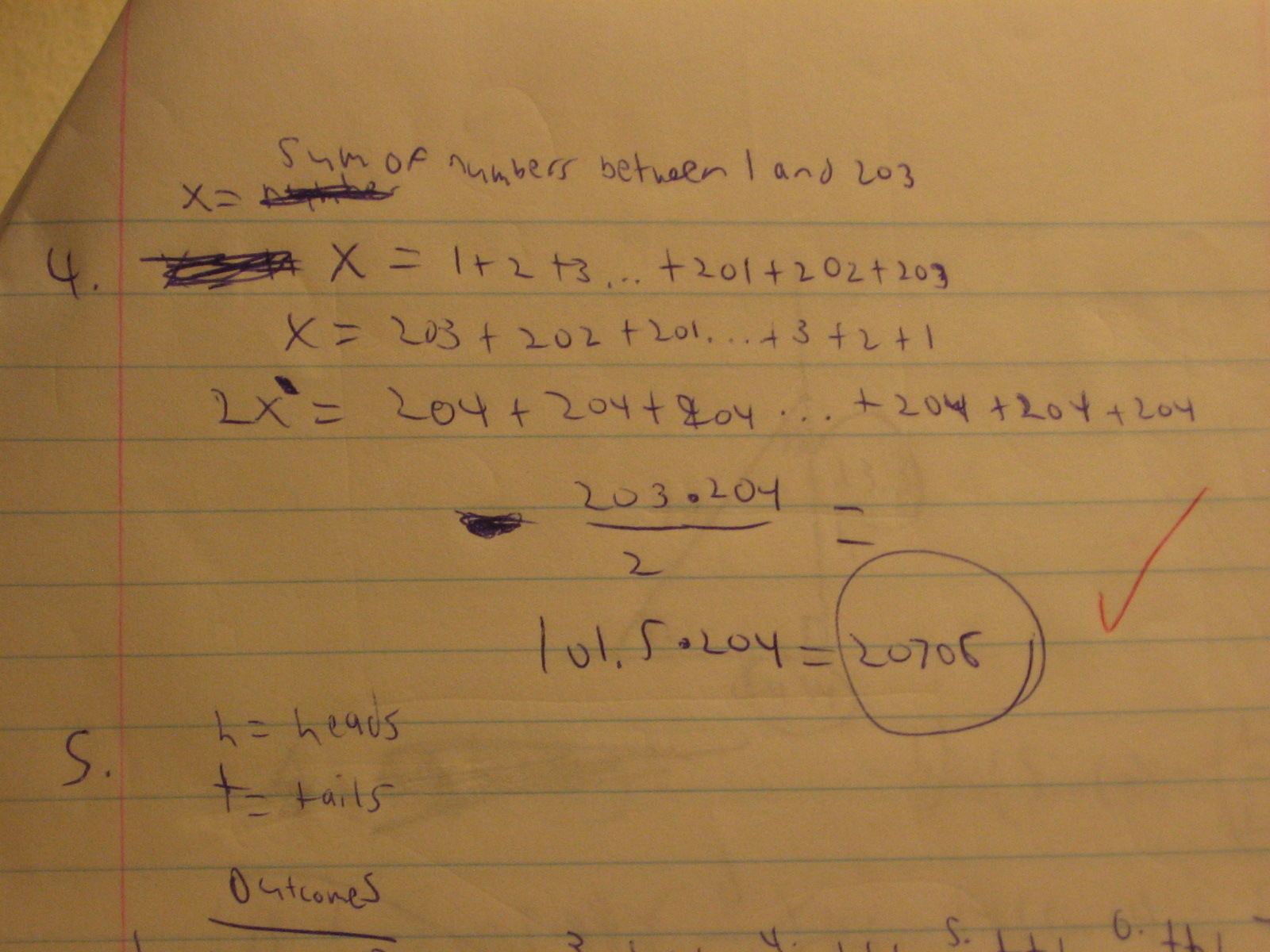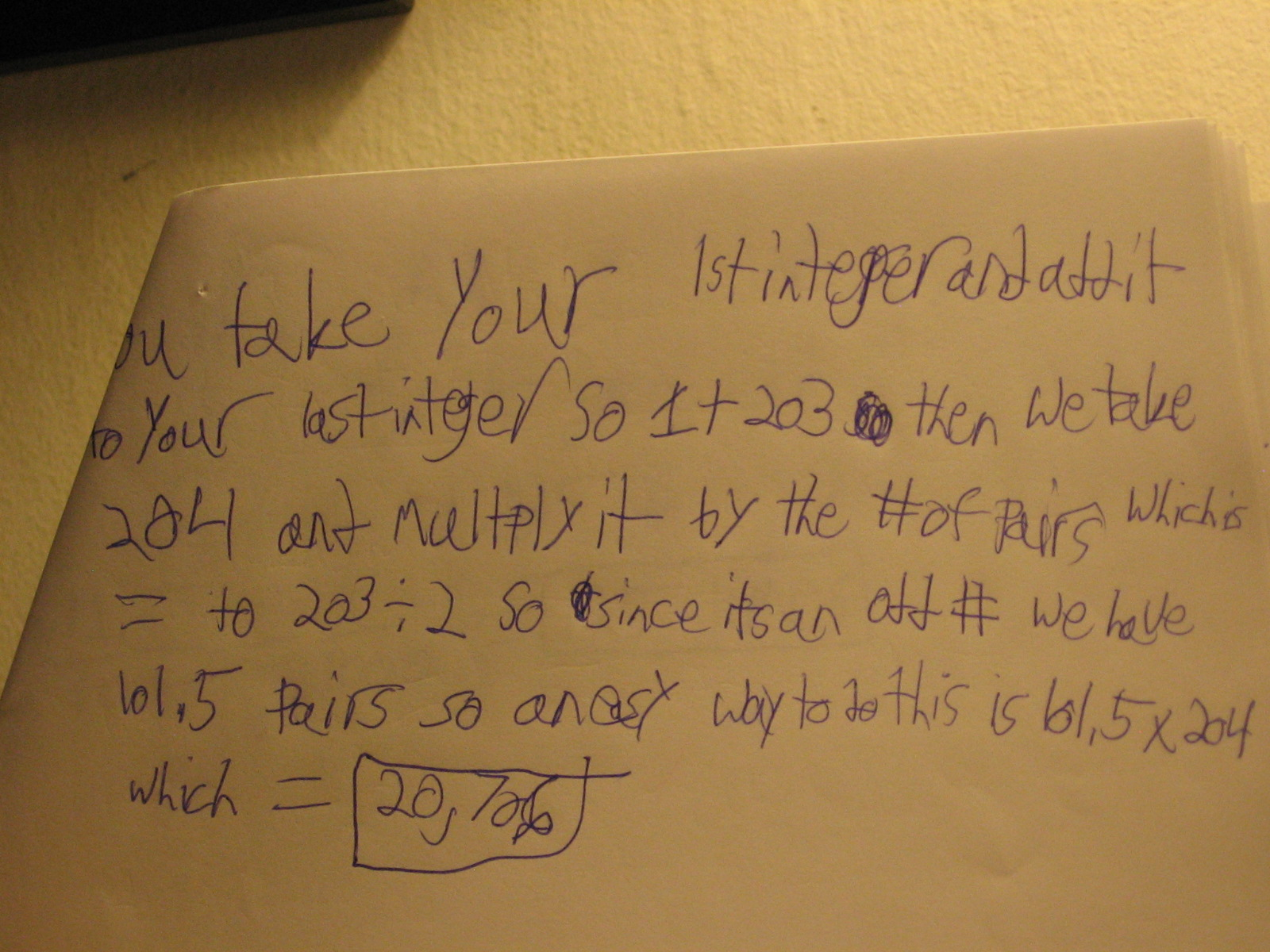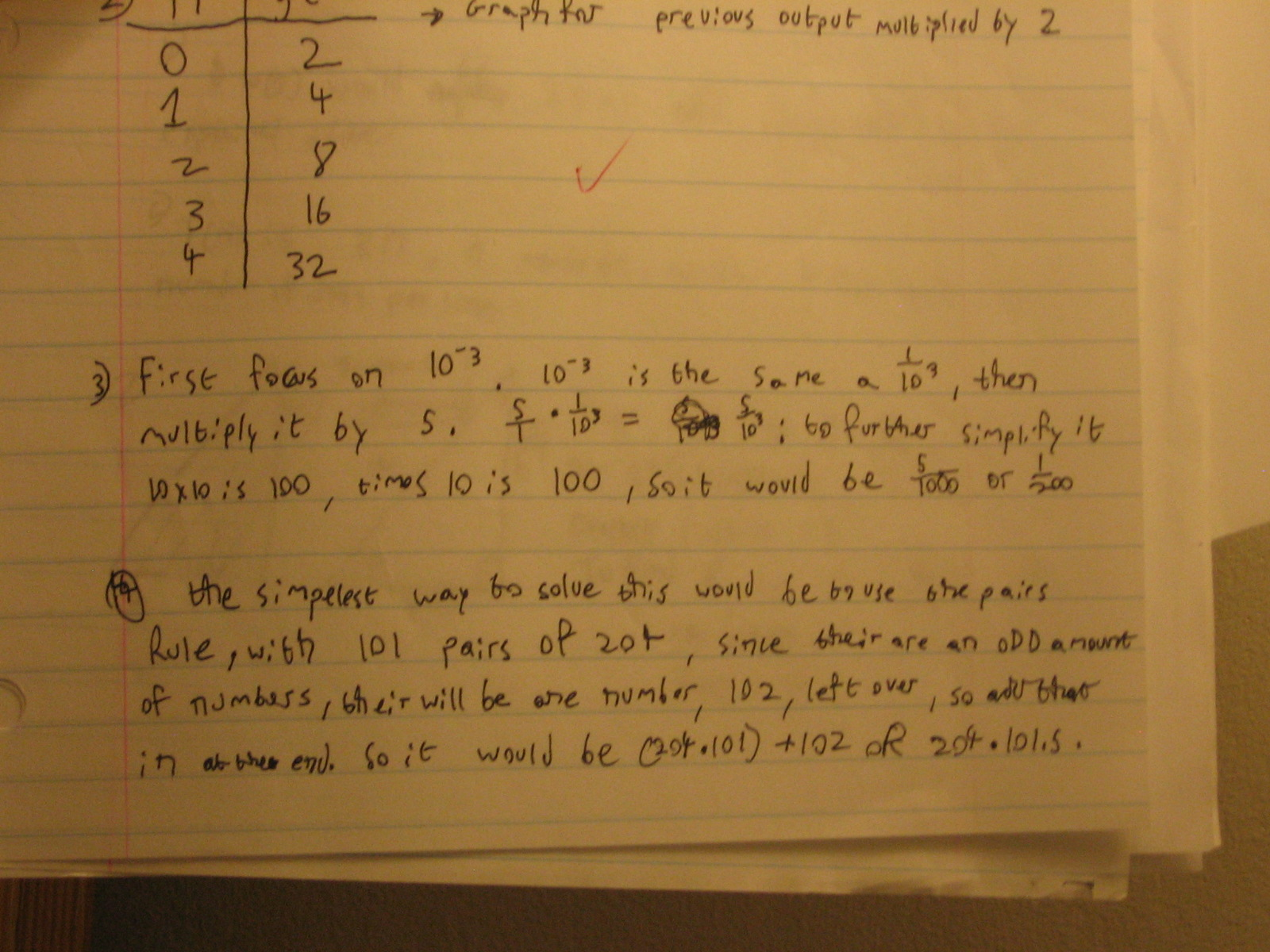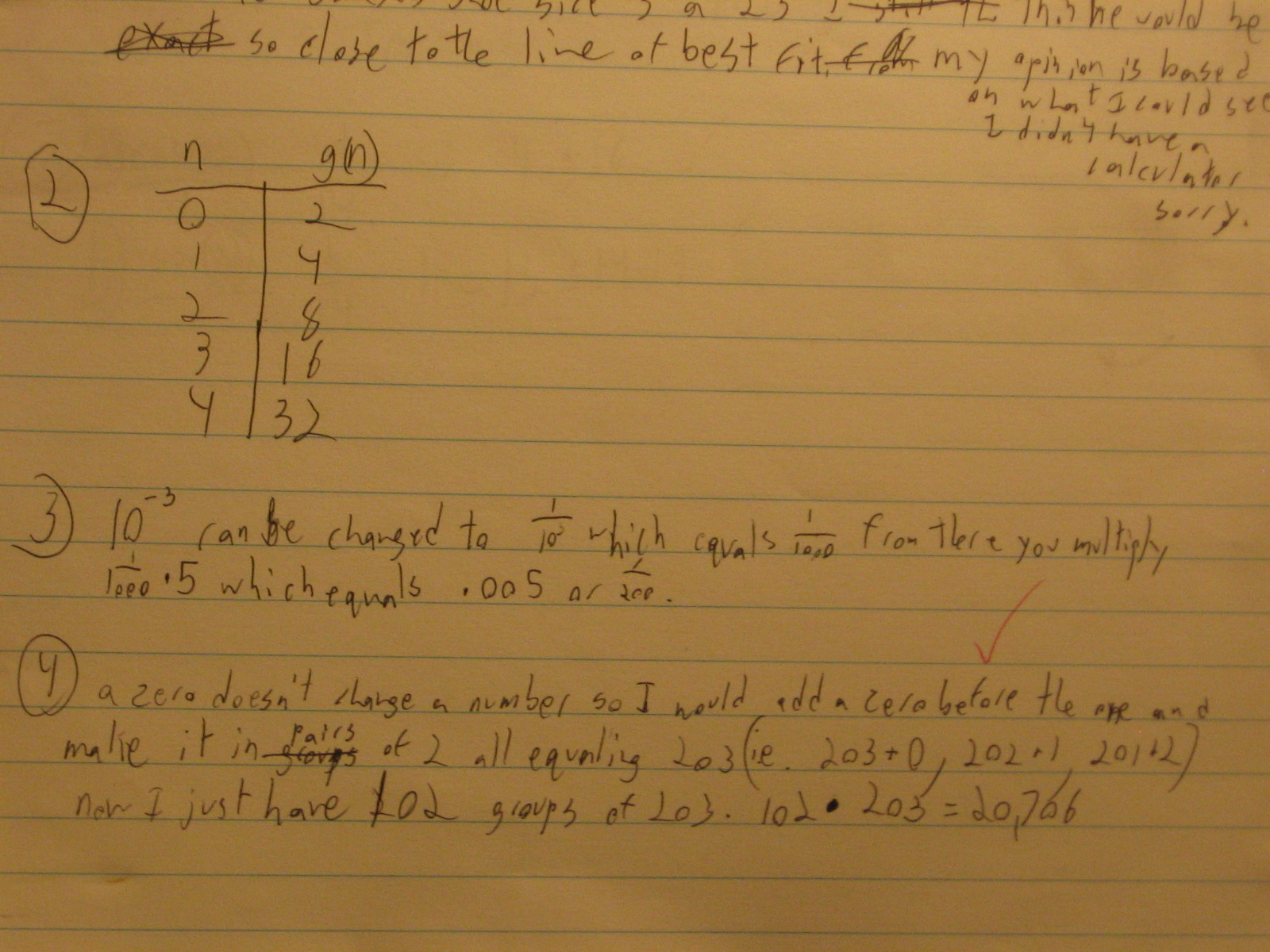Categories

## Arithmetic Series and Subtracting Signed NumbersWhat feedback would you write on this kid’s paper? Why?

(Thanks, KN!)

Categories

## Sum of an Infinite SeriesHere are the results from the 59 students who answered this question on an exam:How would you give feedback to the students who wrote “infinity”?

Imagine that you were to give feedback to the students who wrote “-3/7.” What feedback would you give?

Categories

## Four approaches to adding up all integers from 1 to 203.

We’re looking at #4 here, guys: “Use a non-calculator shortcut to add up all the integers from 1 to 203.”Are these differences significant? Which is most appropriate to introduce to students first? Do you introduce multiple techniques explicitly to your classes? What does this student work indicate about the way this class was taught?

Or, talk about whatever you like.

Categories

## Odd Pairing

“Add up all of the numbers between 1 and 100.”

Easy, once you realize that (1, 100) make a pair whose sum is 101, (2, 99) make another such pair, etc.

“Add up all of the numbers between 1 and 101.”

Harder to make sense of, because there’s an odd number of integers that you’re summing, so they can’t be paired off evenly.

I’ve got (at least) pieces of student work that show (at least) 3 different ways of handling an odd number of integers, as in the problem above.

In the comments below, try to catch ’em all. I’ll update this post with the actual student work tomorrow.

Update: For pictures of student work on this, see the next day’s post.